Author: Oscar Cronquist Article last updated on September 08, 2020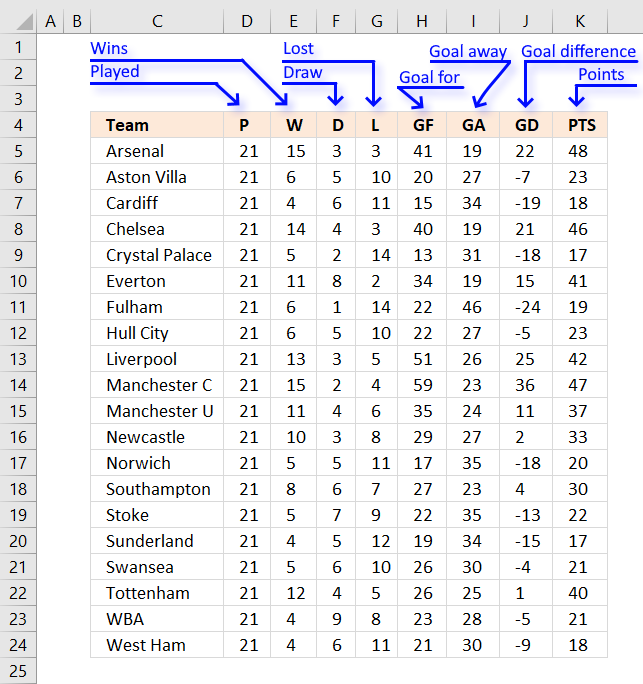This article demonstrates how to sort a data set by multipe columns using the SORTBY function, Ecel Table and Excel formulas.  The example data used here is a table from the Premiere league, see image above.

How are soccer teams ranked in Premier League?Teams are ranked by total points, then goal difference, and then goals scored. At the end of each season, the club with the most points is crowned champion. If points are equal, the goal difference and then goals scored determine the winner. If still equal, teams are deemed to occupy the same position. Source: Wikipedia

Points = Wins * 3 + Draws

Goals for -> Goals made by the team

Goals away or against -> Goals made by the opponent team

Goal difference = Goal for - Goal against

This means that we need to sort the table displayed in the image above first by column K (PTS), second by column J (GD - Goal difference), and third by column H (GF - Goals for).

### Sort by multiple columns using an Excel Table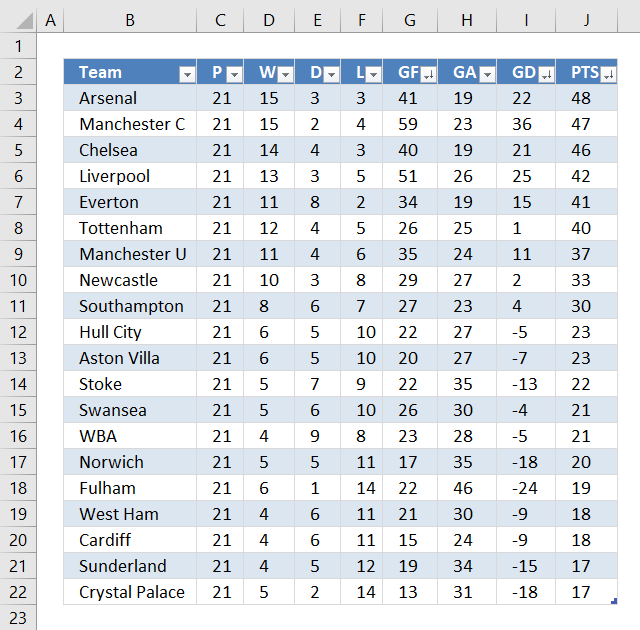The Excel Table is capable of sorting multiple columns in a specified order ascending and descending and which order sorting columns by. The image above shows buttons next to each column header name, next to the black triangle is a down-pointing arrow indicating that the column is sorted.

The image shows three down-pointing arrows, column J (PTS), I (GD) and G (GF). The table is sorted record by record based on the values in column J, I and G and in that order.

#### Convert data to an Excel Table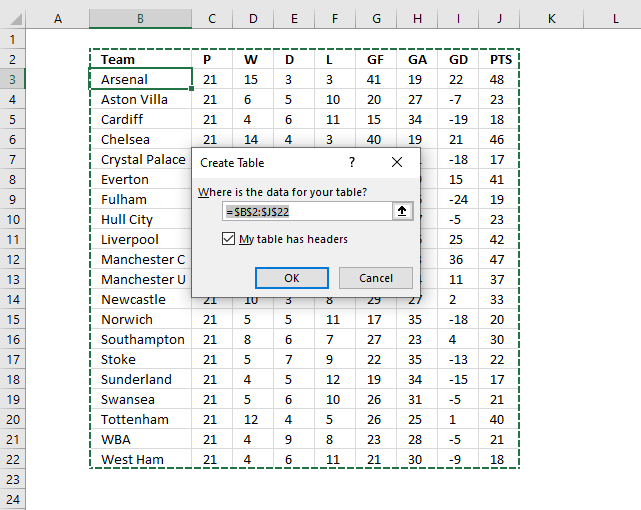1. Select any cell in the data set.
2. Press CTRL + T to open the "Create Table" dialog box. See image above.
3. Click OK button.

#### Apply custom sort to an Excel Table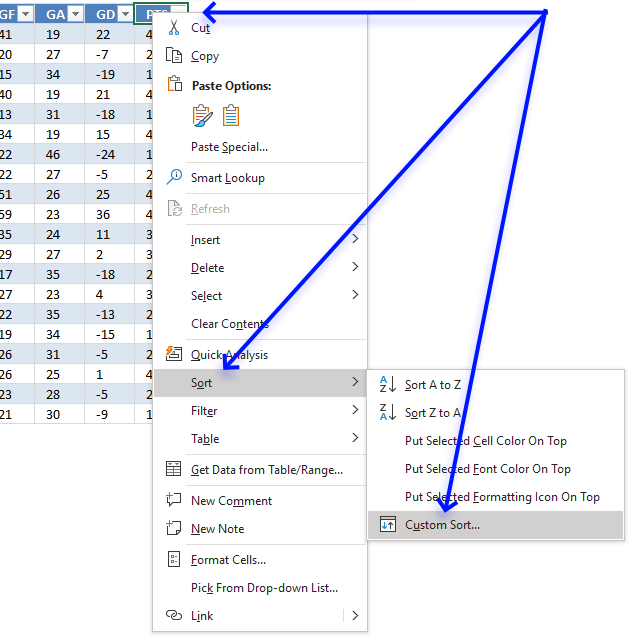1. Right-click on any cell in the Excel Table.
2. A pop-up menu appears, see image above. Click or hover over "Sort".
3. Another pop-up menu shows up, click on "Custom Sort...".
4. A dialog box appears.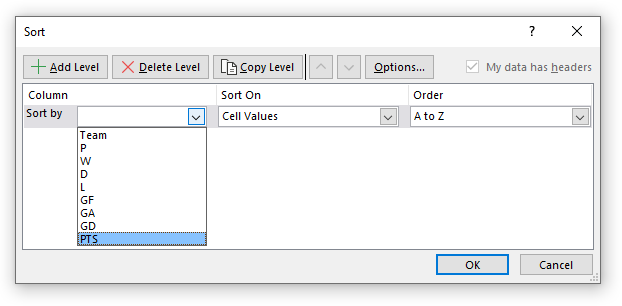5. Click on the first drop-down list, see image above.
6. Click on the column header that you want to sort first by. I chose PTS.
7. The "A to Z" drop-down list changed to "Smallest to Largest". Click on it to expand and see all drop-down list values.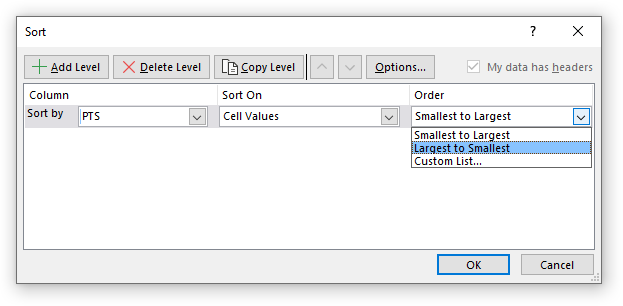8. Click on "Largest to Smallest".
9. Click on "Add Level" button.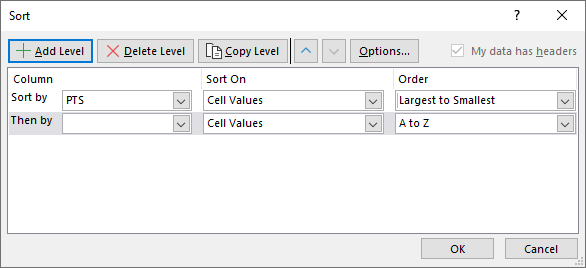10. Now select the next column header name to sort by. I chose "GD".
11. Repeat with the next column header name (GF).
12. Click OK button to apply settings.### Sort by multiple columns using the SORTBY function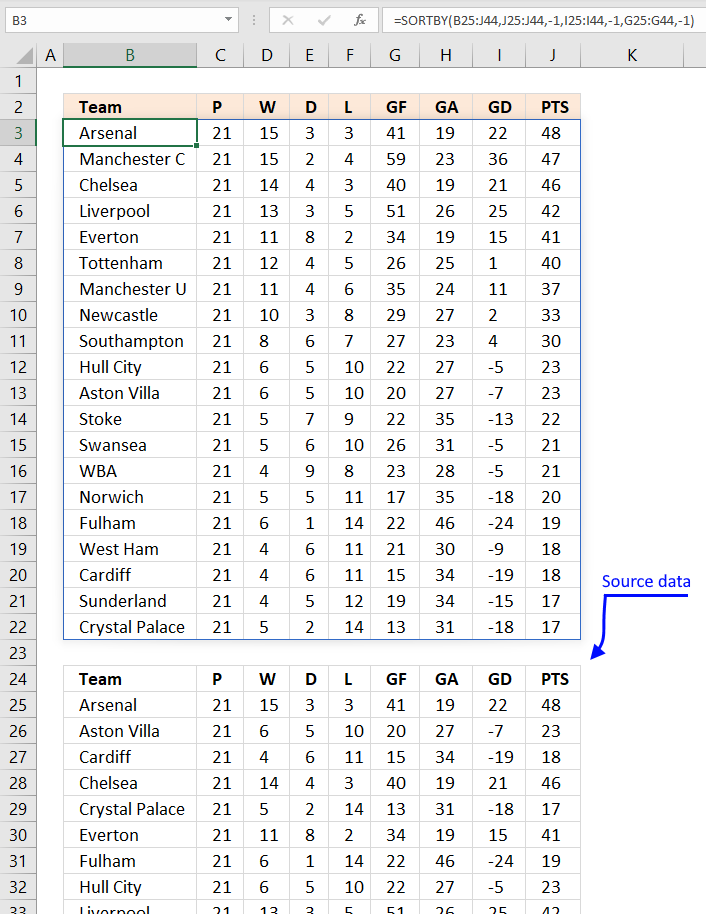Formula in cell B3:

=SORTBY(B25:J44, J25:J44, -1, I25:I44, -1, G25:G44, -1)

The SORTBY function is available for Excel 365 subscribers, it has the following arguments:

SORTBY(array, by_array1, [sort_order1], [by_array2] , [sort_order2],…)

array - An array or cell reference to be sorted

by_array1 - An array or cell reference to be sorted on, this does not have to be a column in the array range. It can be a column outside the array range as well.

[sort_order1] - 1 for ascending, -1 for descending. Default value is -1 (ascending).

The arguments in brackets are optional arguments.

The formula above is a dynamic array formula meaning it spills values across columns and rows automatically.

Spilling is something dynamic array formulas do when they extend to adjacent cells as far as needed. The #SPILL! error is returned if a cell is not empty meaning dynamic array formulas will never overwrite populated cells.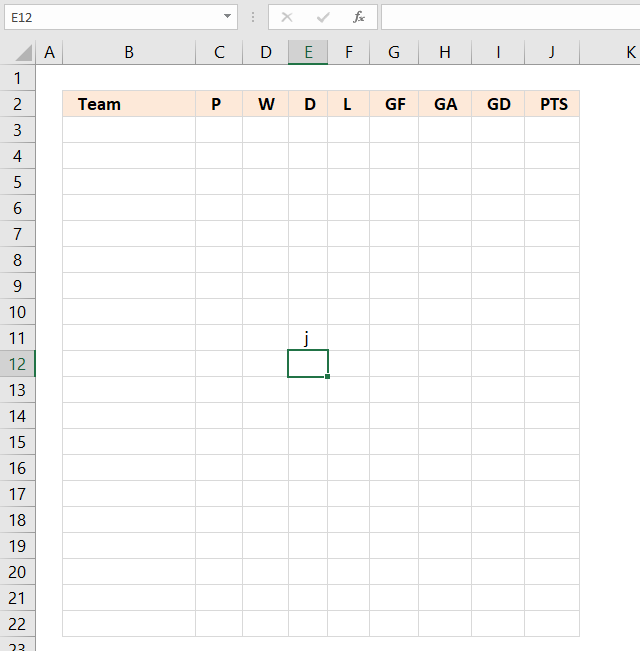As soon as you delete the contents of populated cells the dynamic array formula automatically extends to neighboring cells.

### Sort by multiple columns using formula (Previous Excel versions)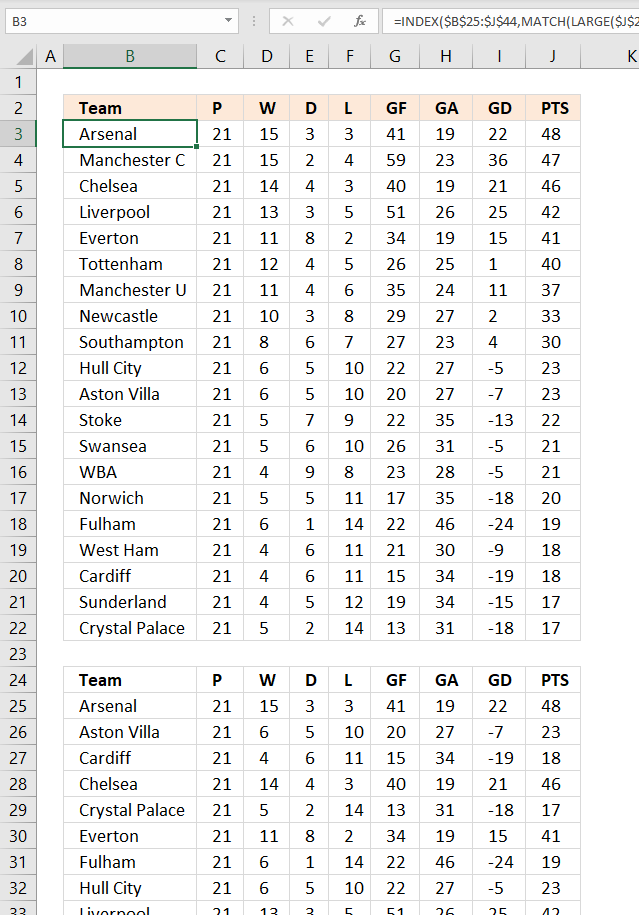The following formula sorts the table keeping the rows intact just like the above examples, the data source is in cell range B25:J44.

Array formula in cell B3:

=INDEX(\$B\$25:\$J\$44, MATCH(LARGE(\$J\$25:\$J\$44+(\$I\$25:\$I\$44+ABS(MIN(\$I\$25:\$I\$44)))/1000+\$G\$25:\$G\$44/10000, ROWS(\$A\$1:A1)), \$J\$25:\$J\$44+(\$I\$25:\$I\$44+ABS(MIN(\$I\$25:\$I\$44)))/1000+\$G\$25:\$G\$44/10000, 0), COLUMNS(\$A\$1:A1))

#### How to enter an array formula in cell B3

1. Copy the above formula.
2. Double click on cell B3, a prompt appears in cell B3.
3. Paste formula to the cell.
4. Press and hold CTRL and SHIFT simultaneously.
5. Press Enter once.
6. Release all keys.

The formula begins and ends now with a curly bracket, like this: {=array_formula}

#### How to copy array formula

1. Click on cell B3 to select it.
2. Press CTRL + c to copy cell.
3. Select cell range C3:J3.
4. Paste to cell range C3:J3. CTRL + v.
5. Select cell range B4:J22.
6. Paste to cell range B4:J22. CTRL + v.

### Explaining formula in cell B3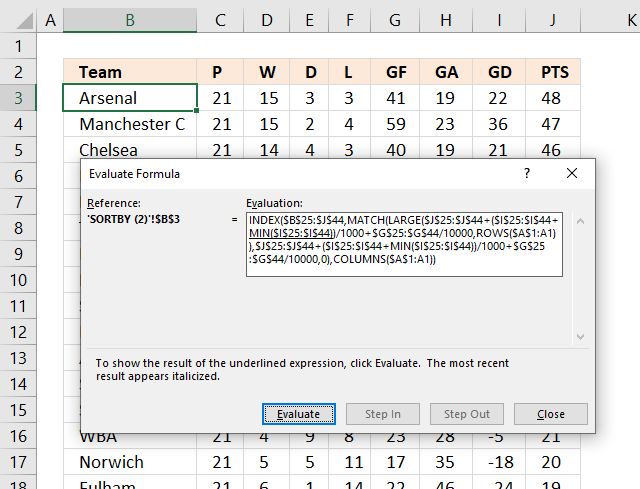The "Evaluate Formula" tool allows you to troubleshoot and examine formulas in great detail. Go to tab "Formulas" on the ribbon. Click on "Evaluate Formula" button.

A dialog box appears, see image above. Click the Evaluate button repeatedly to move through the calculation step by step.

#### Step 1 - Get points from column J

The following cell reference gets the points from the table.

\$J\$25:\$J\$44

returns

{48; 47; 46; 42; 41; 40; 37; 33; 30; 23; 23; 22; 21; 21; 20; 19; 18; 18; 17; 17}

#### Step 2 - Adjust negative numbers (GD) and divide with 1000

The Goal difference number can be negative, to adjust for that I am using the MIN function. The MIN function finds the smallest number in the cell range.

The ABS function removes the sign.

(\$I\$25:\$I\$44+MIN(\$I\$25:\$I\$44))/1000

becomes

({22; 36; 21; 25; 15; 1; 11; 2; 4; -5; -7; -13; -5; -5; -18; -24; -9; -19; -15; -18}+ABS(MIN(\$I\$25:\$I\$44)))/1000

becomes

({22; 36; 21; 25; 15; 1; 11; 2; 4; -5; -7; -13; -5; -5; -18; -24; -9; -19; -15; -18}+ABS(MIN({22; 36; 21; 25; 15; 1; 11; 2; 4; -5; -7; -13; -5; -5; -18; -24; -9; -19; -15; -18})))/1000

becomes

({22; 36; 21; 25; 15; 1; 11; 2; 4; -5; -7; -13; -5; -5; -18; -24; -9; -19; -15; -18}+ABS(-24))/1000

becomes

({22; 36; 21; 25; 15; 1; 11; 2; 4; -5; -7; -13; -5; -5; -18; -24; -9; -19; -15; -18}+24)/1000

becomes

{46; 60; 45; 49; 39; 25; 35; 26; 28; 19; 17; 11; 19; 19; 6; 0; 15; 5; 9; 6}/1000

and returns

{0.046; 0.06; 0.045; 0.049; 0.039; 0.025; 0.035; 0.026; 0.028; 0.019; 0.017; 0.011; 0.019; 0.019; 0.006; 0; 0.015; 0.005; 0.009; 0.006}

#### Step 3 - Divide with 10000

We can't just add the goal difference to the points, that would destroy the sorting.

\$G\$25:\$G\$44/10000

becomes

{41; 20; 15; 40; 13; 34; 22; 22; 51; 59; 35; 29; 17; 27; 22; 19; 26; 26; 23; 21}/10000

and returns

{0.0041; 0.002; 0.0015; 0.004; 0.0013; 0.0034; 0.0022; 0.0022; 0.0051; 0.0059; 0.0035; 0.0029; 0.0017; 0.0027; 0.0022; 0.0019; 0.0026; 0.0026; 0.0023; 0.0021}

#### Step 4 - Add numbers

Step 4 adds the results from step to step 3.

\$J\$25:\$J\$44+(\$I\$25:\$I\$44+MIN(\$I\$25:\$I\$44))/1000+\$G\$25:\$G\$44/10000

becomes

{48; 47; 46; 42; 41; 40; 37; 33; 30; 23; 23; 22; 21; 21; 20; 19; 18; 18; 17; 17} + {0.046; 0.06; 0.045; 0.049; 0.039; 0.025; 0.035; 0.026; 0.028; 0.019; 0.017; 0.011; 0.019; 0.019; 0.006; 0; 0.015; 0.005; 0.009; 0.006} + {0.0041; 0.002; 0.0015; 0.004; 0.0013; 0.0034; 0.0022; 0.0022; 0.0051; 0.0059; 0.0035; 0.0029; 0.0017; 0.0027; 0.0022; 0.0019; 0.0026; 0.0026; 0.0023; 0.0021}

and returns

{48.0501; 47.062; 46.0465; 42.053; 41.0403; 40.0284; 37.0372; 33.0282; 30.0331; 23.0249; 23.0205; 22.0139; 21.0207; 21.0217; 20.0082; 19.0019; 18.0176; 18.0076; 17.0113; 17.0081}

#### Step 5 - Calculate k-th largest number

The LARGE function extracts the k-th largest number in an array or cell range. LARGE(array, k)

LARGE(\$J\$25:\$J\$44+(\$I\$25:\$I\$44+ABS(MIN(\$I\$25:\$I\$44)))/1000+\$G\$25:\$G\$44/10000, ROWS(\$A\$1:A1))

becomes

LARGE({48.0501; 47.062; 46.0465; 42.053; 41.0403; 40.0284; 37.0372; 33.0282; 30.0331; 23.0249; 23.0205; 22.0139; 21.0207; 21.0217; 20.0082; 19.0019; 18.0176; 18.0076; 17.0113; 17.0081}, ROWS(\$A\$1:A1))

The ROWS function calculates the number of rows in a given cell reference. The cell reference in this case grows when we copy the cell and paste to cells below. This is possible if we use both an absolute and relative cell reference. This will  return a new value in each cell.

LARGE({48.0501; 47.062; 46.0465; 42.053; 41.0403; 40.0284; 37.0372; 33.0282; 30.0331; 23.0249; 23.0205; 22.0139; 21.0207; 21.0217; 20.0082; 19.0019; 18.0176; 18.0076; 17.0113; 17.0081}, ROWS(\$A\$1:A1))

becomes

LARGE({48.0501; 47.062; 46.0465; 42.053; 41.0403; 40.0284; 37.0372; 33.0282; 30.0331; 23.0249; 23.0205; 22.0139; 21.0207; 21.0217; 20.0082; 19.0019; 18.0176; 18.0076; 17.0113; 17.0081}, 1)

and returns

48.0501

#### Step 6 - Find relative position

The MATCH function calculates the relative position of a given value in a cell range or an array. MATCH(lookup_value, lookup_array, [match_type])

MATCH(LARGE(\$J\$25:\$J\$44+(\$I\$25:\$I\$44+ABS(MIN(\$I\$25:\$I\$44)))/1000+\$G\$25:\$G\$44/10000, ROWS(\$A\$1:A1)), \$J\$25:\$J\$44+(\$I\$25:\$I\$44+ABS(MIN(\$I\$25:\$I\$44)))/1000+\$G\$25:\$G\$44/10000, 0)

becomes

MATCH(48.0501, \$J\$25:\$J\$44+(\$I\$25:\$I\$44+ABS(MIN(\$I\$25:\$I\$44)))/1000+\$G\$25:\$G\$44/10000, 0)

becomes

MATCH(48.0501, {48.0501; 47.062; 46.0465; 42.053; 41.0403; 40.0284; 37.0372; 33.0282; 30.0331; 23.0249; 23.0205; 22.0139; 21.0207; 21.0217; 20.0082; 19.0019; 18.0176; 18.0076; 17.0113; 17.0081}, 0)

and returns 1.

#### Step 7 - Return value

The INDEX function returns a value based on a specified row and optional column number.

INDEX(\$B\$25:\$J\$44, MATCH(LARGE(\$J\$25:\$J\$44+(\$I\$25:\$I\$44+ABS(MIN(\$I\$25:\$I\$44)))/1000+\$G\$25:\$G\$44/10000, ROWS(\$A\$1:A1)), \$J\$25:\$J\$44+(\$I\$25:\$I\$44+ABS(MIN(\$I\$25:\$I\$44)))/1000+\$G\$25:\$G\$44/10000, 0), COLUMNS(\$A\$1:A1))

becomes

INDEX(\$B\$25:\$J\$44, 1, COLUMNS(\$A\$1:A1))

The COLUMNS function returns the number of columns in a given cell range. This will make the INDEX function return the correct value in each cell.

INDEX(\$B\$25:\$J\$44, 1, COLUMNS(\$A\$1:A1))

becomes

INDEX(\$B\$25:\$J\$44, 1, 1)

and returns "Arsenal" in cell B3.